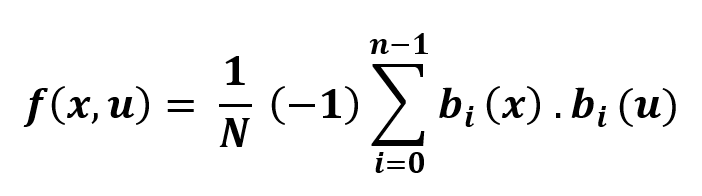# Python | Fast Walsh Hadamard Transform

Fast Walsh Hadamard Transform, is an Hadamard ordered efiicient algorithm to compute the Walsh Hadamard transform (WHT). Normal WHT computation has N = 2m complexity but using FWHT reduces the computation to O(n2). The FWHT requires O(n logn) additions and subtraction operations. It is a divide and conquer algorithm which breaks down the WHT recursively.sympy.discrete.transforms.fwht( ) : It can perform Walsh Hadamard Transform (WHT). This method uses Hadamard sequence ordering.
Automatically the sequence is padded with zero to the right because the radix-2 FWHT requires the sample point number as a power of 2.

```
Parameters :
-> seq : [iterable] sequence on which WHT is to be applied.

Returns :
```

Example #1 :

 `# import sympy  ` `from` `sympy ``import` `fwht ` ` `  `# sequence  ` `seq ``=` `[``23``,  ` `       ``56``,  ` `       ``12``,  ` `       ``555``] ` ` `  `# hwht ` `transform ``=` `fwht(seq) ` `print` `(``"Transform  : "``, transform) `

Output :

`Transform  :  [646, -576, -488, 510]`

Example #2 :

 `# import sympy  ` `from` `sympy ``import` `fwht ` ` `  `# sequence  ` `seq ``=` `[``15``, ``21``, ``13``, ``44``] ` ` `  `# hwht ` `transform ``=` `fwht(seq) ` `print` `(``"Transform  : "``, transform) `

Output :

```Transform  :  [93, -37, -21, 25]
```

My Personal Notes arrow_drop_upCheck out this Author's contributed articles.

If you like GeeksforGeeks and would like to contribute, you can also write an article using contribute.geeksforgeeks.org or mail your article to contribute@geeksforgeeks.org. See your article appearing on the GeeksforGeeks main page and help other Geeks.

Please Improve this article if you find anything incorrect by clicking on the "Improve Article" button below.

Article Tags :

Be the First to upvote.

Please write to us at contribute@geeksforgeeks.org to report any issue with the above content.